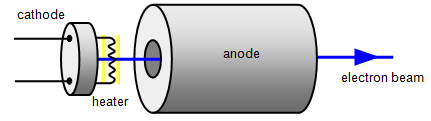# Electron gun and cathode rays

In the thermionic diode a beam of electrons moves a cross the tube and hits the positive anode.

If a hole is made in the anode the electrons will go through it and a stream of electrons will emerge on the other side. This device is called an electron gun.

Usually the anode is made cylindrical as shown in the diagram. A high voltage, often as high as 3000 V, is connected between the cathode and the anode and this accelerates the electrons to a high speed – around 30 000 000 m/s or about 1/10 of the speed of light!The beam of electrons is called a cathode ray because it starts from the cathode.

Experiments with cathode rays show that they have the following properties:

1. Cathode rays are beams of fast moving electrons
2. Increasing the heater voltage heats the cathode more and so it gives out more electrons. This makes the beam stronger (brighter)
3. Increasing the anode voltage makes the electrons move faster by giving them more energy
4. Cathode rays do not pass through glass or metal
5. Cathode rays travel in straight lines in a vacuum
6. Cathode rays travel in circles in magnetic fields
7. Cathode rays travel in parabolas in electric fields
8. Cathode rays have a limited range in gases. This range increases as the energy of the electrons in the beam increases.

You can work out the energy and speed of the electrons using the following formulae.

Since: Energy = charge x voltage       the electrical energy of one electron is:    Energy = eV

Where e is the charge on the electron and V is the accelerating voltage, the voltage difference between the cathode and the anode. But this is converted in to the kinetic energy of the electron (˝mv2) where m is the mass of one electron and v is its speed.

Therefore: ˝mv2 = eV and so the speed of the electron can be found by using the formula:

Electron speed (v) = (2eV/m)1/2

Example problem
The mass of an electron is 9x10-31 kg and its charge 1.6x10-19 C.
For an accelerating voltage of 3000 V:

Electron speed = [2x1.6x10-19x3000/9x10-31]1/2 = 3.3x107 m/s = 33 000 000 m/s

A VERSION IN WORD IS AVAILABLE ON THE SCHOOLPHYSICS CD